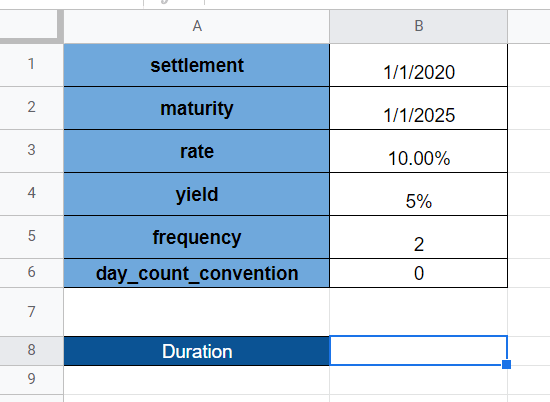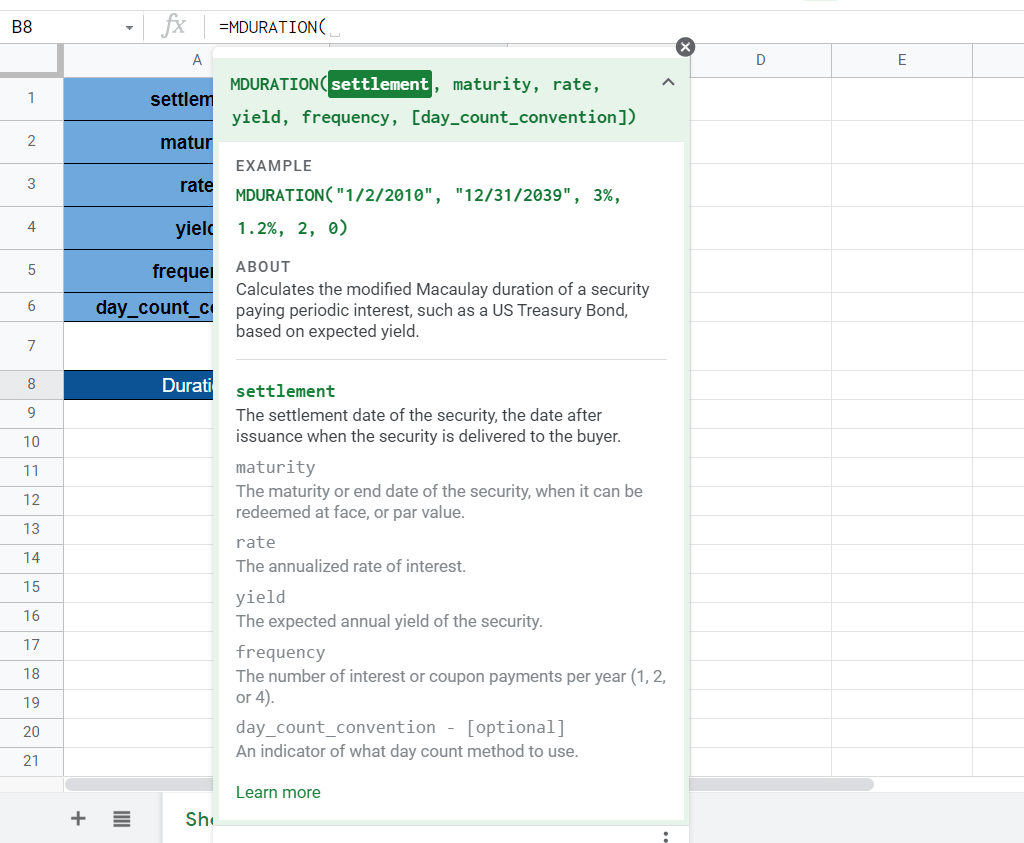# How To Use MDURATION Function in Google Sheets

The MDURATION function in Google Sheets is useful when you need to return the modified Macaulay duration of a security that pays periodic interest.

The modified Macaulay duration calculates how much the duration changes for each percentage change in the yield. Specifically, this computation helps measure how much a change in the interest rate impacts the price of the security.

The rules for using the `MDURATION` function in Google Sheets are as follows:

• The function requires several arguments to describe the security. The user of the function must indicate the settlement date, maturity date, the annualized rate of interest, the expected annual yield, and the frequency of coupon payments.
• The function then outputs the value of the modified Macaulay duration of the given security.

Let’s take a look at a brief example!

In this example we have a security bond that was delivered to the buyer on January 1st, 2021. We expect to fully redeem this bond five years later on January 1st, 2026. This particular bond has an annualized interest of 10% and an annual yield to maturity of 5%, with semiannual payments. We would like to know how many years it will take before the bond’s cash flow equals the amount paid for the bond. Additionally, we want to find out how the duration will change given a 1% increase in the yield to maturity.

With the `MDURATION` function, we can solve the modified Macaulay duration of this particular security. In this case, the modified Macaulay duration is useful because of the changing yield to maturities. To calculate the change in duration given a 1% increase, we just need to get the difference between the modified duration and the Macaulay duration. We can obtain the latter by using the `DURATION` function.

Now that we’ve seen an example of when to use the `MDURATION` function, let’s explore how we can write the function down and later work on an actual sample spreadsheet.

## The Anatomy of the MDURATION Function

So the syntax (the way we write it) of the `MDURATION` function is as follows:

```=MDURATION(settlement, maturity, rate, yield, frequency, [day_count_convention])
```

Let’s dissect this function’s arguments in order to understand what each of these terms means:

• = the equal sign is how we start any function in Google Sheets.
• MDURATION() is our `MDURATION` function. It computes the modified Macaulay duration of a given security paying periodic interest.
• settlement refers to the security’s settlement date, or when the security is given to the buyer.
• maturity refers to the date when the security is redeemed.
• rate is the annualized rate of interest.
• yield refers to the expected annual yield of the security.
• frequency is the number of interest payments per year.
• day_count_convention is an optional argument that indicates which day count method to use.

## A Real Example of Using MDURATION Function

In this section, we’ll be looking into a real example of the `MDURATION` function being used in a Google Sheets spreadsheet.To get the value in cell B8, we just need to use the following formula:

```=MDURATION(B1, B2, B3, B4, B5, B6)
```

You can make a copy of the spreadsheet above using the link I have attached below.

We can also use the `MDURATION` function in Google Sheets to compare the modified Macaulay duration of various bonds. For example, we can have two bonds with slightly different annual yields, as seen in the table below. Using the `MDURATION` values, we can see that bond A has a shorter time to maturity.If you’re prepared to try out the `MDURATION` function in Google Sheets, let’s start writing it ourselves!

## How to Use MDURATION Function in Google Sheets

1. To start using the `MDURATION` function, let’s first select the cell we’ll be placing our results in. In this example, we’ll start with cell B8.2. After that, simply type the equal sign ‘=‘ to begin our function, followed by ‘MDURATION(‘ to indicate which function to use.
3. A tooltip box appears with a guide on how to use the `MDURATION` function. If necessary, we can click on the arrow on the top-right-hand corner of the box to minimize it.4. Now that we’ve added our function name, the next step we need to take is typing in our arguments. In this instance, all our arguments are laid out in order in column B.5. After typing out the right cells, simply hit the Enter key on your keyboard to evaluate the function. In this example, our modified Macaulay duration is 4.05 years!This step-by-step guide should be all you need to start using `MDURATION` in Google Sheets. In summary, it’s a simple way to forecast how long your securities will take before you receive back the amount paid for the given security.

You can certainly use the `MDURATION` functions in Google Sheets together with the many other Google Sheets functions available to create great spreadsheets that help your financial computations. Most importantly, do make sure to subscribe to our newsletter to be the first to know about the latest Google Sheets guides and tutorials from us.Our goal this year is to create lots of rich, bite-sized tutorials for Google Sheets users like you. If you liked this one, you'd love what we are working on! Readers receive ✨ early access ✨ to new content.

##### You May Also Like## How to Create a Combo Chart in Google Sheets

A combo chart combines visual points of two or more charts to present information in one cohesive and…## How to Create a Geographical Map Chart in Google Sheets

The Geographical Map Chart in Google Sheets is useful when you need to visualize geographic data. This type…## How to Perform Polynomial Regression in Google Sheets

Performing polynomial regression in Google Sheets is useful when you need a more complex model of the relationship…## How to Use Query With Importrange in Google Sheets

The Query with Importrange in Google Sheets is useful if you want to pull the exact data that…